#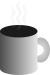Coffee SpaceListen:

## Hash Functions Part 2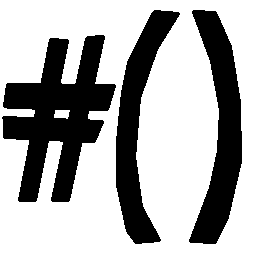Preview Image

In a previous post I wrote about hash functions. I have since had some ideas regarding this and have incremented on some further algorithms to help address the distribution out in the far-end of the hashing.

The focus of this work has been to distribute the key over the entire hash space whilst maintaining reasonable performance. As far as I'm aware, this is somewhat uncharted territory.

### Performance

Firstly we can look at the performance values (everything beyond function 13 is new):

func ms col=8 col=16 col=24 last=24
cust1 2945 16777200 16776960 16773120 16777215
cust2 3236 16777001 16773120 16711680 16777215
cust3 3879 16776960 16711680 15902144 16777215
cust4 3715 16776960 16711680 15741061 16777215
cust5 3596 16776960 16711680 15730195 16777215
cust6 3313 16776960 16711680 15728390 16777215
cust7 3076 16776960 16711680 15632352 16777215
cust8 2968 16776960 16711680 15728624 16777215
cust9 3497 16776960 16711680 6155480 16777215
cust10 3509 16776960 16711680 6138576 16777215
cust11 3136 16776960 16711680 7083260 16777215
cust12 2954 16776960 16711680 5915585 16777215
cust13 2974 16776960 16711680 5915585 16777215
cust14 3835 16776960 16754467 16512407 16773211
cust15 7014 16776960 16711680 10634670 14805065
cust16 4237 16777099 16775953 16768834 16777066
cust17 3823 16776960 16711680 6248433 15886338
cust18 7815 16776960 16711680 6248433 6606591
cust19 6720 16776960 16711680 6248433 6425758
cust20 6865 16776960 16711680 6248421 6605339

As you can see, we now look at the last three bytes collision space to get an idea of how well we distribute our values over the entire hash.

### Function 14

This is my initial attempt at multi-byte hashing. It's quite convoluted and doesn't reach the end of the hash particularly well.

```0001 /* Initial implementation of multi-byte hashing */
0002 char* hash_cust14(char* s, char* d, int n){
0003   /* Copy s into d until either d filled or s length found */
0004   int l = -1;
0005   while(++l < n && s[l]) d[l] = s[l];
0006   /* If s too long, merge with existing bytes */
0007   while(s[l]) d[l % n] += s[l++];
0008   /* Finally do multi-byte merge over safe hash string */
0009   for(int x = 0; x < n;) d[x % n] = d[x % n] - (d[++x % n] << 3) + l;
0010   return d;
0011 }```

Performance: This is not too bad all things considered. It's well within the expected range.

Collisions: As you can see, there are still a significant number of collisions at the end of the hash and we lose all benefits at the start of the hash.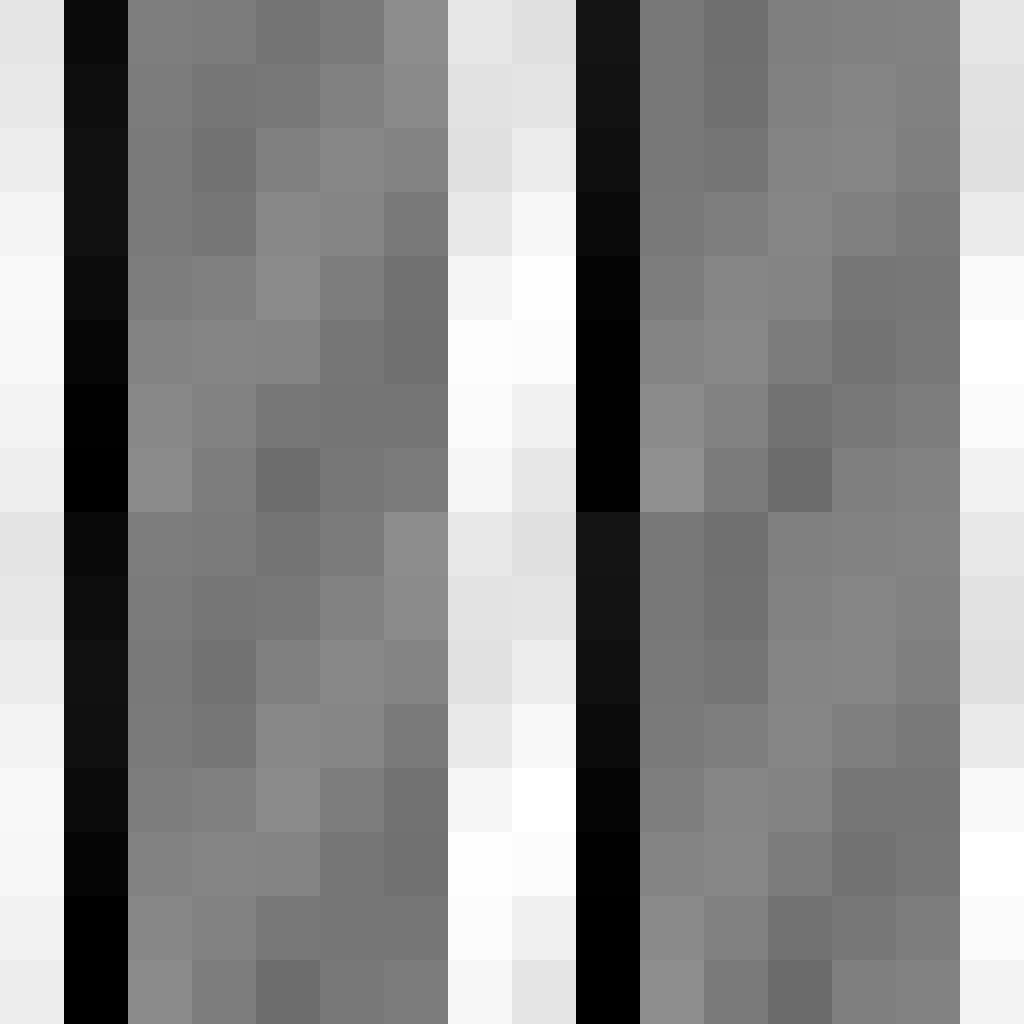One byte

As you can see, the one byte distribution is terrible.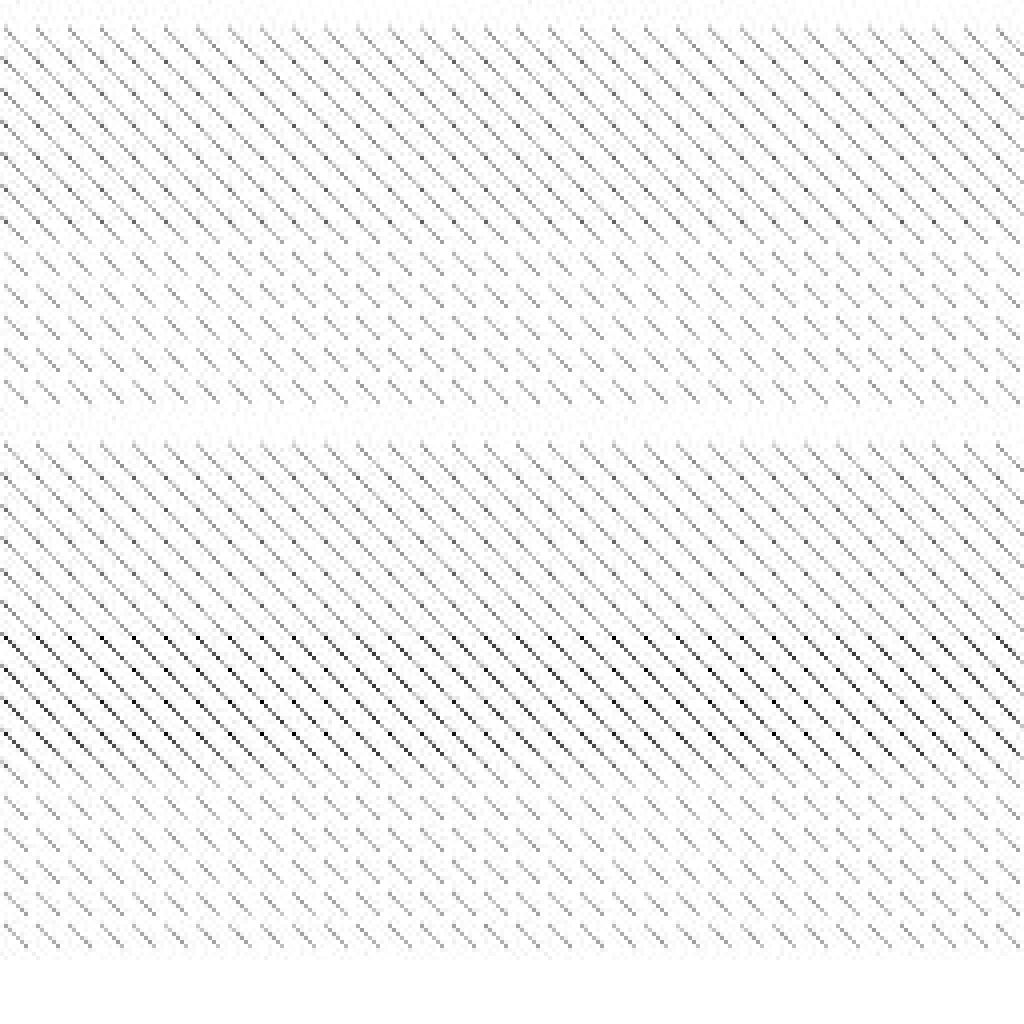Two bytes

The two byte distribution is even worse.Three bytes

The three byte distribution is even worse than the two byte distribution.Last three bytes

As you can see, the last three bytes are barely touched at all.

### Function 15

The idea here was to introduce an additional hashing process at the end. Again, it's quite complicated and doesn't work well.

```0012 /* Full coverage, weak distribution, long run time */
0013 char* hash_cust15(char* s, char* d, int n){
0014   /* Copy s into d until either d filled or s length found */
0015   int l = -1;
0016   while(++l < n && s[l]) d[l] = s[l];
0017   /* Back hash */
0018   for(int x = l; s[++x];) d[x % n] ^= s[x] + l;
0019   /* Forward hash */
0020   for(char* z = d; z < d + n - 1;)
0021     *(int16_t*)z = (*(int16_t*)z << 3) ^ *(int16_t*)z ^ (d[(int)(z - d) % 2] ^= *z++);
0022   return d;
0023 }```

Performance: The performance is not great. It does on the other hand begin to achieve the goal.

Collisions: The collisions are certainly much better than the previous function, but still not ideal.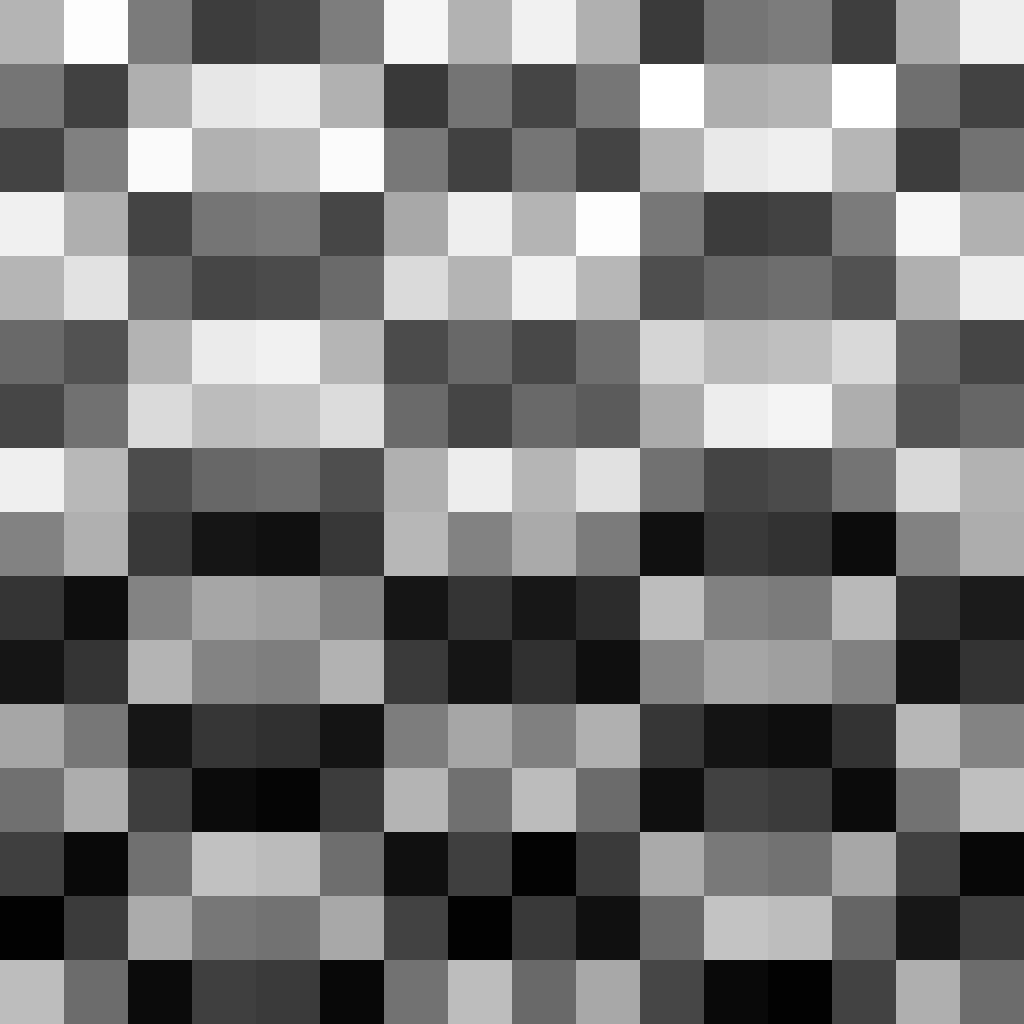One byte

One byte looks alot better.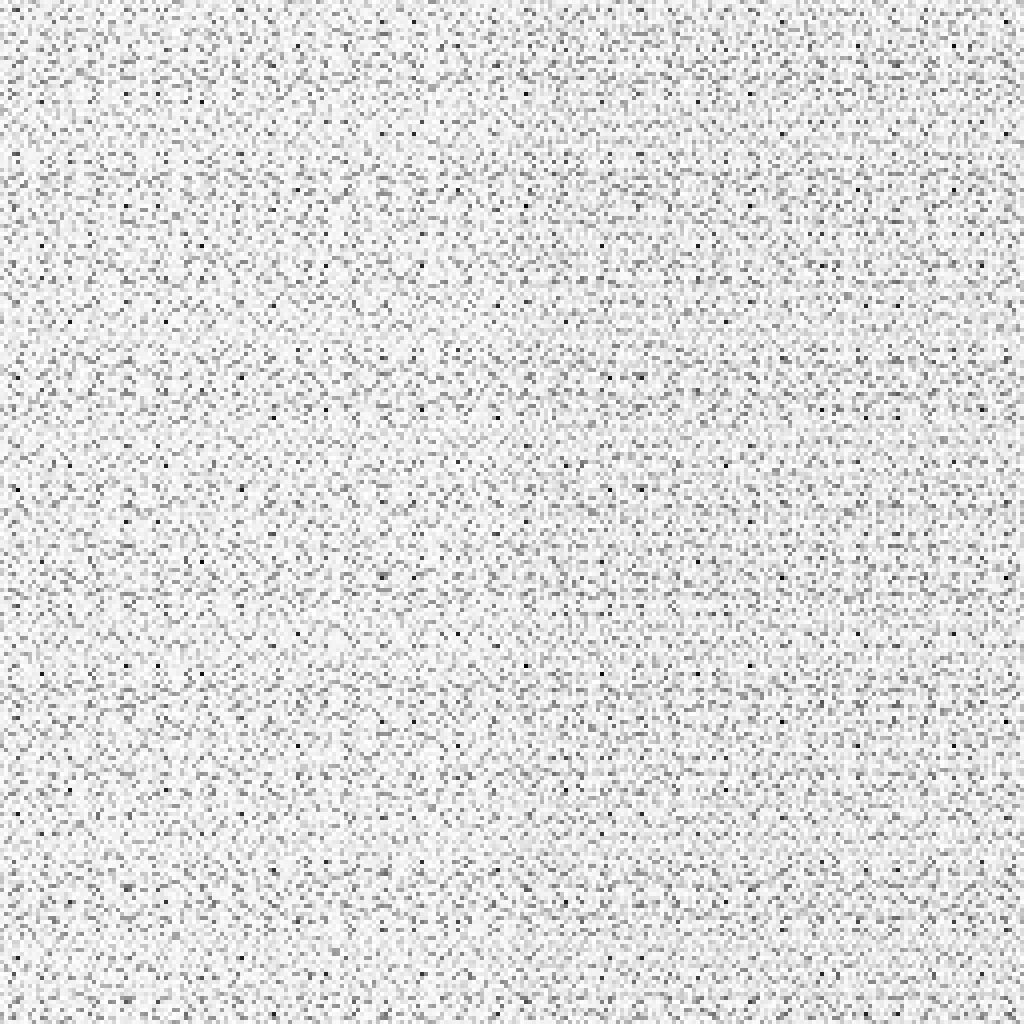Two bytes

Two byte distribution again looks better than the previous function, but it is clearly still highly predictable.Three bytes

The three byte distribution is clearly predictable, but is at least represented.Last three bytes

The last three bytes are really not well distributed at all.

### Function 16

The attempt here was to experiment with single byte hashing. It did not go well, but it was at least fast.

```0024 /* Faster with terrible distribution */
0025 char* hash_cust16(char* s, char* d, int n){
0026   memset(d, 0b10101010, n);
0027   /* Loop hash */
0028   int l = 0;
0029   while(*s) d[l % n] ^= *s++ ^ ++l;
0030   /* Forward hash */
0031   for(int x = 1; x < n; ++x) d[x] ^= (*d += ~d[d[x] % n] ^ l);
0032   return d;
0033 }```

Performance: Performance is really quite good here.

Collisions: The collision space is exceptionally awful.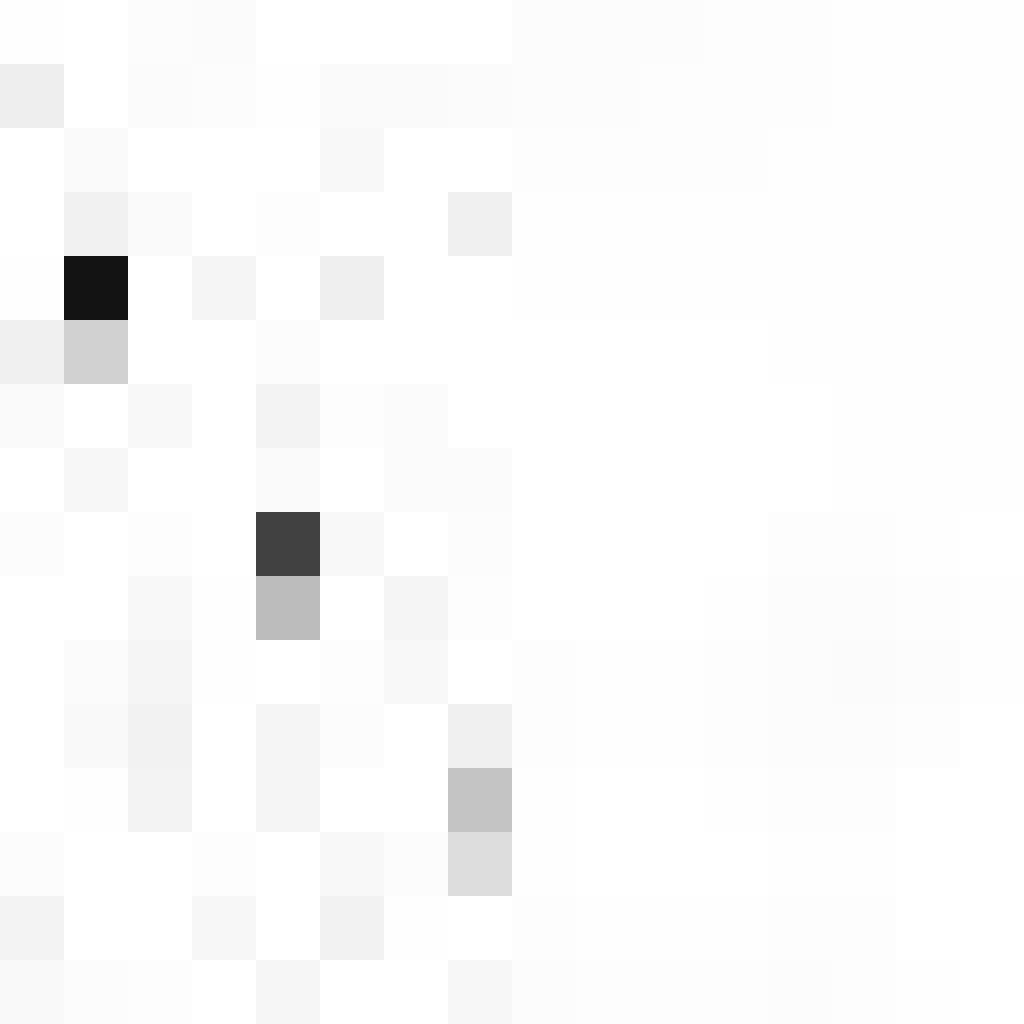One byte

One byte is barely spread, and it only gets worse.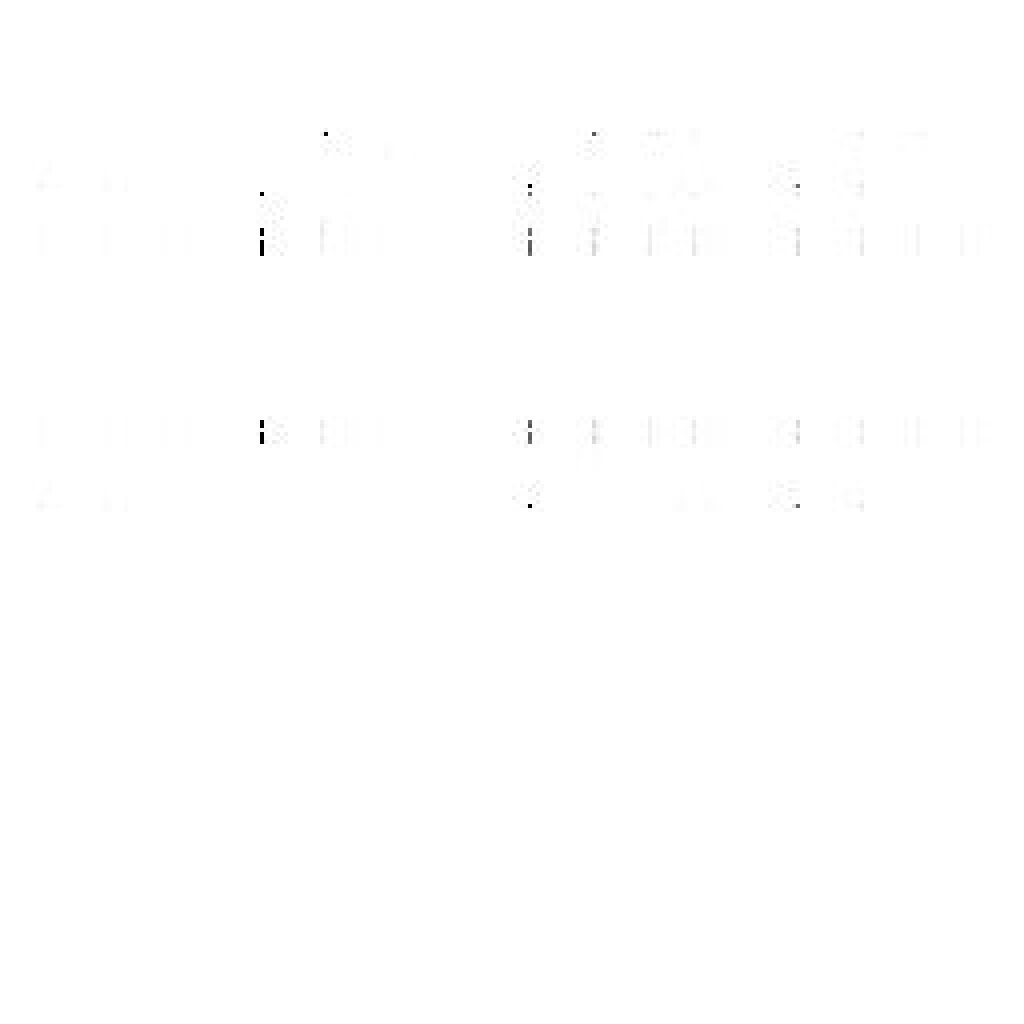Two bytes

Two bytes clearly follows the pattern.Three bytes

Three byte distribution is not represented.Last three bytes

The last three bytes are also not represented.

### Function 17

Single byte hashing was a failure, so I went back to multi-byte hashing. This experiment consists of two parses and an initial hash seed.

```0034 /* Better wide distribution, better speed */
0035 char* hash_cust17(char* s, char* d, int n){
0036   memset(d, 0b10101010, n);
0037   for(int x = 0; *s;)
0038     *(uint32_t*)d = *(int16_t*)(d + 2) + (*(int32_t*)d << 5) + *(int32_t*)d + (d[x++ % n] ^= *s++);
0039   for(int x = 3; ++x < n;) d[x] = d[x] ^ d[x % 4] ^ d[x - 1];
0040   return d;
0041 }```

Performance: It runs incredibly fast. If based on speed per gains, this is the best implementation.

Collisions: The distribution is really quite reasonable. We see clearly that each area of the hash has at least some reorientation.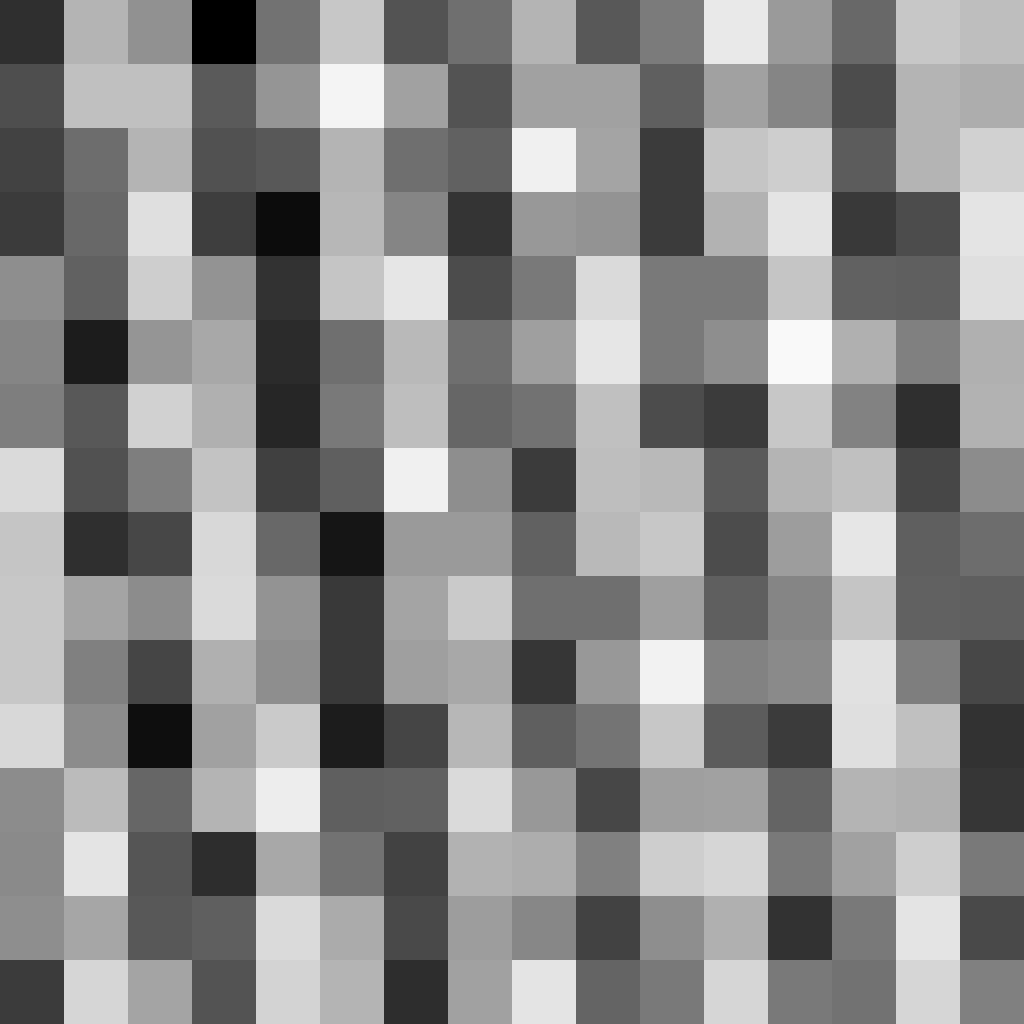One byte

The one byte distribution is not bad at all.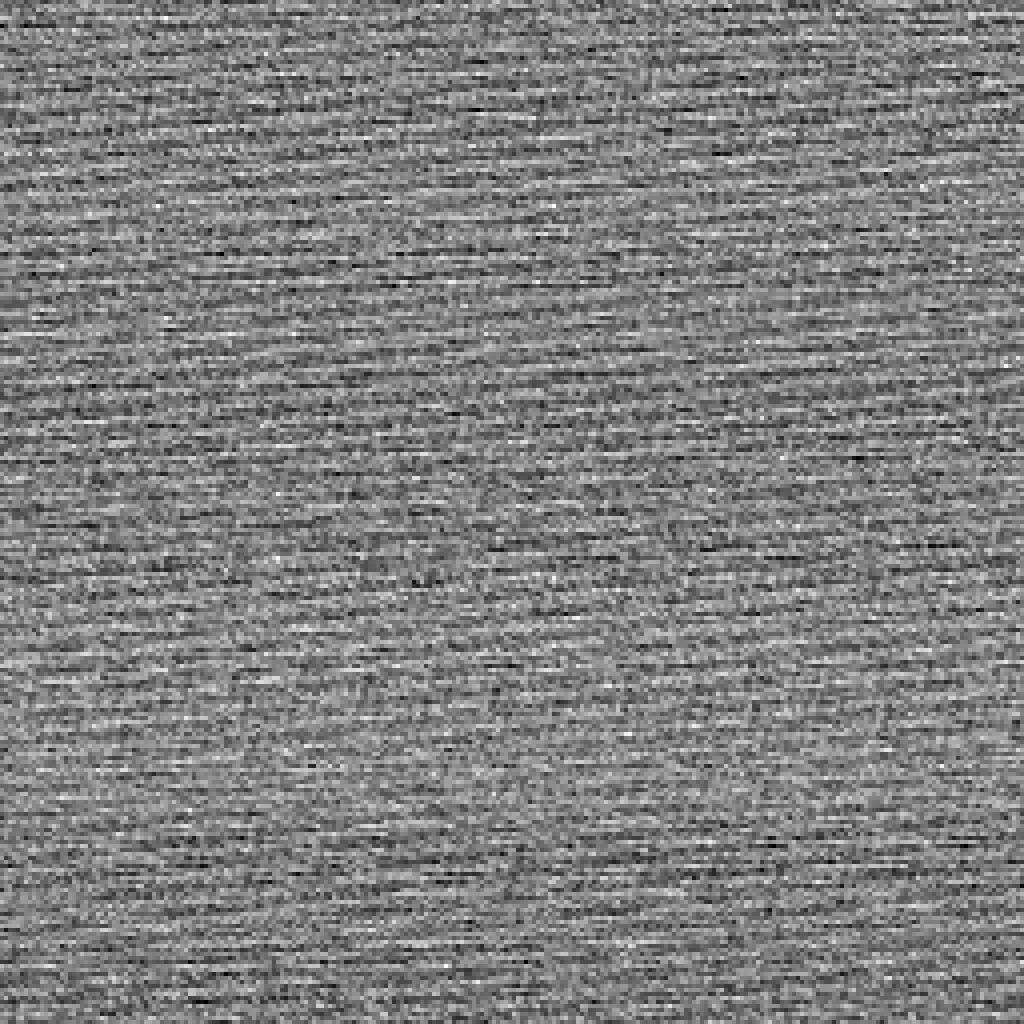Two bytes

The two byte distribution is is really quite nice.Three bytes

The three byte distribution we are starting to see some patterns, but in general it is also really not so bad at all.Last three bytes

The last three bytes have some form of pattern, but the data is at least represented reasonably out here.

### Function 18

With this function I took a step back, re-thought about the problem and then took another crack at it. The major change is the second part of the function, where we essential perform a second run over the hash.

```0042 /* Much better distribution at the cost of computation speed */
0043 char* hash_cust18(char* s, char* d, int n){
0044   memset(d, 0b10101010, n);
0045   for(int x = 0; *s;)
0046     *(uint32_t*)d = *(int16_t*)(d + 2) + (*(int32_t*)d << 5) + *(int32_t*)d + (d[x++ % n] ^= *s++);
0047   for(int x = 3; ++x < n;)
0048     *(int16_t*)(d + x) = (*(int16_t*)(d + x) << 5) ^ *(int16_t*)(d + x) +
0049                          *(int16_t*)(d + (x % 4)) + *(int16_t*)(d + x - 1);
0050   return d;
0051 }```

Performance: One of the heaviest algorithms tested so far, but introduce some nice distribution.

Collisions: This is the first algorithm tested that really tackles the last three bytes (therefore the whole hash) distribution quite well.One byte

One byte is not the best I've seen, but it's passable.Two bytes

The two byte is really not too bad, but acceptable.Three bytes

The three byte pass is not too bad, but pretty good.Last three bytes

The last three bytes are very good. Look at how smooth it is!

### Function 19

Here we improve the distribution and improve the speed marginally.

```0052 /* Slightly better distribution */
0053 char* hash_cust19(char* s, char* d, int n){
0054   memset(d, 0b10101010, n);
0055   for(int x = 0; *s;)
0056     *(uint32_t*)d = *(int16_t*)(d + 2) + (*(int32_t*)d << 5) + *(int32_t*)d + (d[x++ % n] ^= *s++);
0057   for(int x = 3; ++x < n - 1;)
0058     *(int16_t*)(d + x) = (*(int16_t*)(d + x) << 3) ^ *(int16_t*)(d + x) +
0059                          (*(int16_t*)(d + (x % 4)) ^ *(int16_t*)(d + x - 1));
0060   return d;
0061 }```

Performance: Runs slightly faster. This is the fastest three byte implementation so far.

Collisions: This has some of the best multi-byte distributions too.One byte

One byte is okay, but not great.Two bytesThree bytes

Three bytes are nice, a little wavy, meaning there is a little predictability there.Last three bytes

The last three bytes are quite nice, with a small pattern present.

### Function 20

For this implementation we look to reduce the running time a little further.

```0062 /* Reduction in running time, slight reduction in tail-end distribution */
0063 char* hash_cust20(char* s, char* d, int n){
0064   memset(d, 0b10101010, n);
0065   int x = 0;
0066   while(*s)
0067     *(uint32_t*)d = *(int16_t*)(d + 2) + (*(int32_t*)d << 5) +
0068                     *(int32_t*)d       + (d[x++ % n] ^= *s++);
0069   for(; x < n - 1; ++x)
0070     *(int16_t*)(d + x) = (*(int16_t*)(d + x) << 3) ^ *(int16_t*)(d + x) +
0071                          (*(int16_t*)(d + (x % 4)) ^ *(int16_t*)(d + x - 1));
0072   return d;
0073 }```

Performance: On testing, the performance was a little worse.

Collisions: Slightly worse than the previous distribution.One byte

Again, a familiar one byte spread.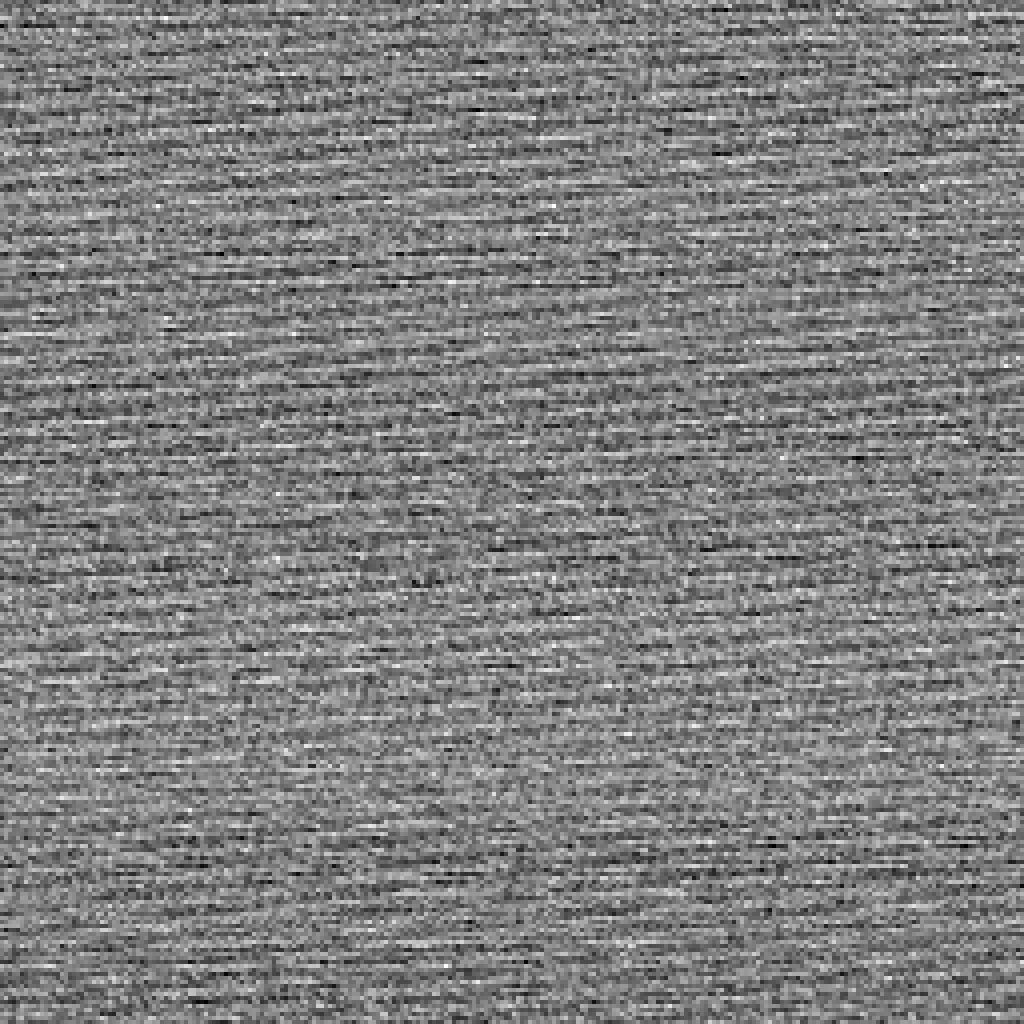Two bytes

Two bytes are also not too bad.Three bytes

Three bytes we see more of the same pattern.Last three bytes

The last three bytes are okay, but not too great.

### Going Further

Function 19 is by far the best function so far for full byte distribution.

It's still not perfect though. I wrote a tool to test it and found the following collision:

```0074 \$ echo "test  " | ./beesum -n 16
0075 C4D5F9C5FB4ACFF95C3E189802AEA902
0076 \$ echo "testtt" | ./beesum -n 16
0077 C4D5F9C5FB4ACFF95C3E189802AEA902```

Future tests should measure the collisions with nearby hashes. This will require some thinking as to exactly how this can be achieved.

Additionally I still aim to make the function work faster, and with single bytes. This will of course require some thinking in order to achieve this.